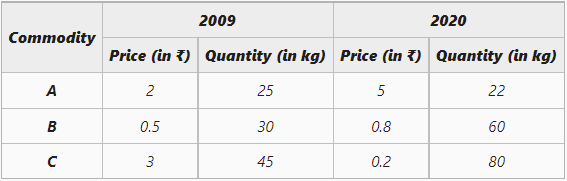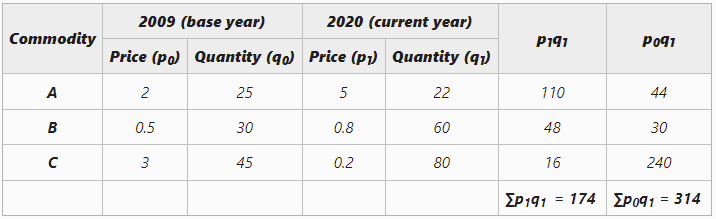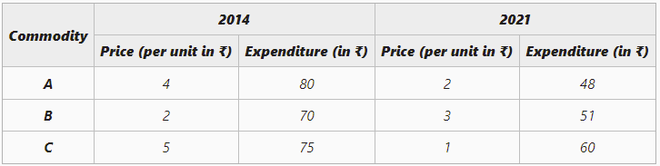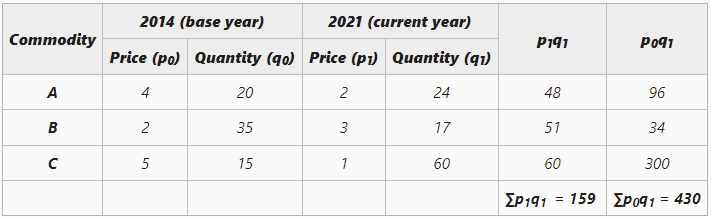GFG App
Open AppBrowser
Continue

# Paasche’s Method of calculating Weighted Index Number

A statistical measure that helps in finding out the percentage change in the values of different variables, such as the price of different goods, production of different goods, etc., over time is known as the Index Number. The percentage change is determined by taking a base year as a reference. This base year is the year of comparison. When an investigator studies different goods simultaneously, then the percentage change is considered the average for all the goods. There are two broad categories of Index Numbers: viz., Simple and Weighted Index Numbers.

According to Spiegel, “An Index Number is a statistical measure designed to show changes in a variable or group of related variables with respect to time, geographic location or other characteristics.”

According to Croxton and Cowden, “Index Numbers are devices for measuring difference in the magnitude of a group of related variables.”

## Paasche’s Method

Weighted Index Numbers can be constructed by using two methods: Weighted Average of Price Relatives Method and Weighted Aggregative Method. Under the Weighted Aggregative Method, different weights are given to different goods according to the quantity bought. There are three methods through which weighted index numbers can be calculated under the Weighted Aggregative Method, viz., Laspeyre’s, Paasche’s, and Fisher’s Method.

The method of calculating Weighted Index Numbers under which the current year’s quantities are used as weights of different items is known as Paasche’s Method. The formula for Paasche’s Price Index is:Here,

P01 = Price Index of the current year

p0 = Price of goods in base year

q1 = Quantity of goods in base year

p1 = Price of goods in the current year

#### Example 1

Based on the following data, construct Paasche’s Price Indices for 2020 with 2009 as the base.#### Solution:= 55.41

Paasche’s Price Index is 55.41

Example 2

Construct a price index number from the following data by using Paasche’s Method.#### Solution:

The formula for constructing Index Numbers through Paasche’s Method requires quantity and the given data contains information about the expenditure of the commodities. We can obtain quantity for the given commodities by dividing total expenditure by the price of each commodity.= 36.97

The Price index using Paasche’s Method is 36.97

My Personal Notes arrow_drop_up